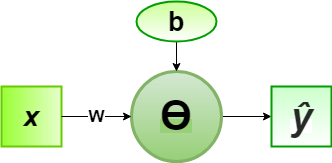# Implementation of Perceptron Algorithm for NOT Logic Gate

In the field of Machine Learning, the Perceptron is a Supervised Learning Algorithm for binary classifiers. The Perceptron Model implements the following function:For a particular choice of the weight vectorand bias parameter, the model predicts outputfor the corresponding input vector.

NOT logical function truth table is of only 1-bit binary input (0 or 1), i.e, the input vectorand the corresponding output0 1
1 0

Now for the corresponding weight vectorof the input vector, the associated Perceptron Function can be defined as:For the implementation, considered weight parameter isand the bias parameter is.

Python Implementation:

 # importing Python library  import numpy as np     # define Unit Step Function  def unitStep(v):      if v >= 0:          return 1     else:          return 0    # design Perceptron Model  def perceptronModel(x, w, b):      v = np.dot(w, x) + b      y = unitStep(v)      return y     # NOT Logic Function  # w = -1, b = 0.5  def NOT_logicFunction(x):      w = -1     b = 0.5     return perceptronModel(x, w, b)     # testing the Perceptron Model  test1 = np.array(1)  test2 = np.array(0)     print("NOT({}) = {}".format(1, NOT_logicFunction(test1)))  print("NOT({}) = {}".format(0, NOT_logicFunction(test2)))

Output:

NOT(1) = 0
NOT(0) = 1


Here, the model predicted output () for each of the test inputs are exactly matched with the NOT logic gate conventional output () according to the truth table.
Hence, it is verified that the perceptron algorithm for NOT logic gate is correctly implemented.

Whether you're preparing for your first job interview or aiming to upskill in this ever-evolving tech landscape, GeeksforGeeks Courses are your key to success. We provide top-quality content at affordable prices, all geared towards accelerating your growth in a time-bound manner. Join the millions we've already empowered, and we're here to do the same for you. Don't miss out - check it out now!

Previous
Next